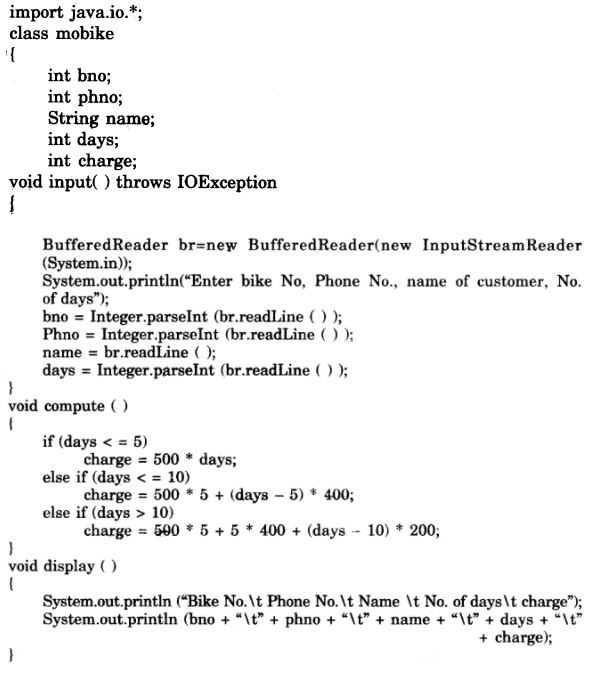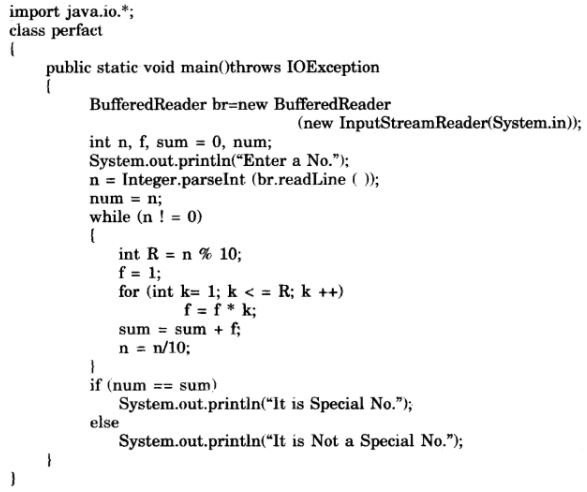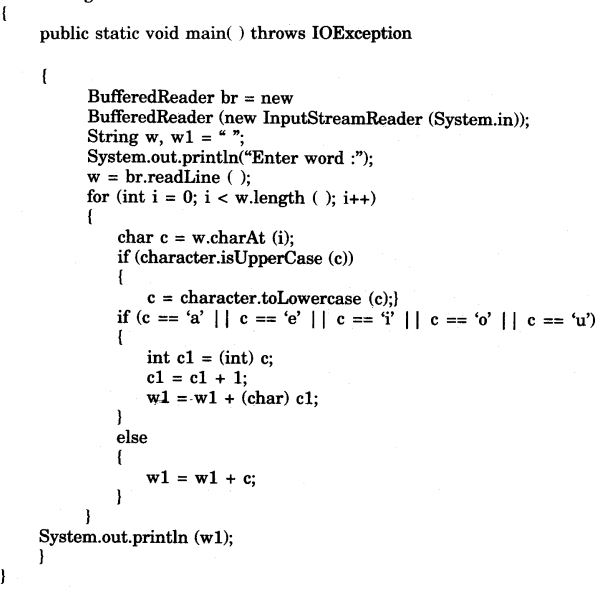Friday, March 24, 2023

# ICSE Computer Applications 2011 Previous Year Question Paper for Class-10

ICSE Computer Applications 2011 Previous Year Question Paper Solved for Class-10 Step by Step Solutions of ICSE Computer Applications 2011 for preparation of board exam. You can get the idea of solving . Visit official website cisce for detail information about ICSE Class-10 Computer Application .

## ICSE Computer Applications 2011 Previous Year Question Paper Solved for Class-10

Try Also other year except Computer Application 2011 Solved Question Paper ICSE Previous Year for practice Because only Computer Application 2011  Solved Question  Paper ICSE  Previous Year is not enough for preparation of upcoming council exam.

### ICSE Computer Applications 2011

(Two Hours)
Answers to this Paper must be written on the paper provided separately.
You will not be allowed to write during the first 15 minutes.
This time is to be spent in reading the question paper.
The time given at the head of this Paper is the time allowed for writing the answers.
This paper is divided into two Sections.
Attempt all questions from Section A and any four questions from Section B.
The intended marks for questions or parts of questions are given in brackets [ ].

### Section ‘A’ (40 Marks)

(ICSE Computer Applications 2011 Previous Year Question Paper Solved for Class-10)

(Attempt all questions)

Question 1:
(a) What is the difference between an object and a class ? 
(b) What does the token ‘keyword’ refer to, in the context of Java ? Give an example for keyword. 
(c) State the difference between entry controlled loop and exit controlled loop. 
(d) What are the two ways of invoking functions ? 
(e) What is the difference between / and % operator ? 

(a)

 Class Object A class is a template or blueprint or layout according to which object of that class are created. An object is an instance of class. A class will not exist in memory An object will exist in memory.

(b)

Keywords are reserved words, which have special meaning to Java compiler. They form the vocabulary of the language Keywords can not be used as identifier. e.g., class, if, char, new, int etc.

(c)

 Entry control loop Exit control loop In these loops the test condition is evaluated before each iteration. In these loops, condition is evaluated after each iteration. This type of loop can not executed, even once if condition is evaluated to false at first time. This type of loops always executed at least once even if the condition is false.

(d)

Two ways to call a function are : 1. Call by value, 2. Call by reference.

(e)

 / operator % operator This is called division operator. This is called modulus operator. This operator calculates quotient of two nos. e.g. : int x = 10; y = 5, z; z = x/y; value of z will be 2. This operator calculates remainder of two nos. int x = 10„ y = 5, z; z = x % y; value of z will be 0. / can operate on integer as well as real nos. % can operate on integer only.

Question 2:
(a) State the total size in bytes, of the arrays a  of char data type and p of float data type. 
(b)

(i) Name the package that contains Scanner class.

(ii) Which unit of the class gets called, when the object of the class is created ? 

(c) Give the output of the following:
String n=”Computer Knowledge”;
String m=”Computer Applications”;
System. out.println(n. substring(0,8). concat(m. substring(9)));
System.out.println(n.ends With(“e”)); 

(d) Write the output of the following :
(i) System.out.println(Character.isUpperCase(‘R’));
(ii) System.out.println(Character.toUpperCase(‘j’); 

(e) What is the role of keyword void in declaring functions ? 

(a) Size of char a is 4 × 2 = 8 Bytes.
Size of float p is 4 × 4 = 16 Bytes.

(b)

(i) Java.util
(ii) Constructor.

(c) Computer Applications
true.

(d)

(i) true
(ii) J.

(e) At the time of function declaration void signify that function has no return type, that means function will not return any value.

Question 3:
(a) Analyse the following program segment and determine how many times the loop will be executed and what will be the output of the program segment ?
int p= 200;
while(true)
{
if(p<100)
break;
p=p-20;
}
System.out.println(p); 

(b) What will be the output of the following code ?
(i) int k=5, j=9;
k+= k++ – ++j +k;
System.out.println(“k=”+k);
System.out.println(“j=”+j); 
(ii) double b =-15.6;
double a = Math.rint(Math.abs(b)); –
System, out. println(“a=”+a); 

(d) Give the prototype of a function search which receives a sentence sentnc and a word wrd and returns 1 or 0 ? 

(e) Write an expression in Java for [latex s=2]z=\frac { 5{ x }^{ 3 }+2y }{ x+y } [/latex]. 

(f) Write a statement each to perform the following task on a string :
(i) Find and display the position of the last space in a string s.
(ii) Convert a number stored in a string variable x to double data type. 

(g) Name the keyword that
(i) informs that an error has occurred in an input I output operation.
(ii) distinguishes between instance variables and class variables. 

(h) What are library classes ? Give an example. 

(i) Write one difference between Linear Search and Binary Search. 

(a) Output : 80.
The loop will be executed 6 times.

(b) (i) k = 5 + 5 – 10 + 6
= 10-10 + 6
= 6.
output : k = 6
j = 10
(ii) a = 16

(c) When more than one constructor are defined in a class provided they differ in their signature, it Is known as constructor overloading.
Example:

public class Demo
{
private int rollNum;
//We are not defining a no-arg constructor here

Demo(int rnum)
{
rollNum = rollNum+ rnum;
}
//Getter and Setter methods

public static void main(String args[])
{
//This statement would invoke no-arg constructor
Demo obj = new Demo();
}
}

Output:

Exception in thread "main" java.lang.Error: Unresolved compilation
problem:The constructor Demo() is undefined

(d) int search (String sentnc, String wrd)

(e) Expression in Java :
z = ((5 * Math.pow (x, 3)) + (2 * y))\(x + y);

(f)

(i) String s = “this is to test”;
int p;
p = s.
lastIndexOf (“ ”);
System.out.println(p);
(ii) String x = “10.2”;
double d;
d = string.valueOf (x);

(g) (i) throws IOException.
(ii) static keyword.

(h) Library classes are pre-defined classes which are provided by Java System. There is thousands of library classes and each class contains various functions. These classes provide an effective support to programmers in developing their programs.
e.g., Java.lang, Java.util

(i)

 Linear Search Binary Search Linear search can be performed on unsorted arrays. Binary search can be performed on only sorted arrays.

### SECTION B (60 Marks)

(ICSE Computer Applications 2011 Previous Year Question Paper Solved for Class-10)

Attempt any four questions from this Section.
The answers in this Section should consist of the Programs in either Blue J environment or any program environment with Java as the base.
Each program should be written using Variable descriptions /Mnemonic Codes so that the logic of the program is clearly depicted.
Flow-Charts and Algorithms are not required.

Question 4:
Define a class called mobike with the following description:
Instance variables /data members:
int bno — to store the bike’s number
int phno — to store the phone number of the customer
String name — to store the name of the customer
int days — to store the number of days the bike is taken on rent
int charge — 4 calculate and store the rental charge
Member methods:
void input() — to input and store the detail of the customer
void compute() — to compute the rental charge.
The rent for a mobike is charged on the following basis:
First five days Rs. 500 per day.
Next five days Rs. 400 per day.
Rest of the days Rs. 200 per day.

void display() — to display the details in the following format:  
Bike No,-   Phone No –   Name – No of Days –  ChargeQuestion 5:

Write a program to input and store the weight of ten people. Sort and display them in descending order using the selection sort techniqueQuestion 6:
Write a program to input a number and print whether the number is a special number or not.
(A number is. said to be a special number, if the sum of the factorial of the digits of the number is same as the original number).
Example : 145 is a special number, because 1!+4!+5! = 1 + 24 + 120 = 145 (Where ! stands for factorial of the number and the factorial value of a number is the product of all integers from 1 to that number, example 5! = 1*2*3*4*5 = 120).Question 7:
Write a program to accept a word and convert it into lowercase if it is in uppercase, and display the new word by replacing only the vowels with the character following it :
Example :
Sample Input : computer
Sample Output : cpmpvtfr 

class changewordQuestion 8:
Design a class to overload a function compared as follows :
(a) void compare(int, int) — to compare two integer values and print the greater of the two integers.
(b) void compare(char, char) — to compare the numeric value of two characters and print the character with higher numeric value.
(c) void compare(String, String) — to compare the length of the two strings and print the longer of the two.Question 9:
Write a menu driven program to perform the following: (Use switch-case statement)
(a) To print the series 0, 3, 7, 15, 24, …… n terms (value of ‘n’ is to be an input by the user).
(b) To find the sum of the series given below :
S = 1/2 + 3/4 + 5/6 + 7/8 + ……… 19/20.}  }  }

-: End of ICSE Computer Application 2011 for Class-10  :-

Thanks

RELATED ARTICLES